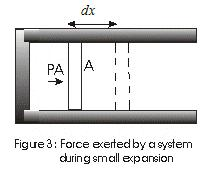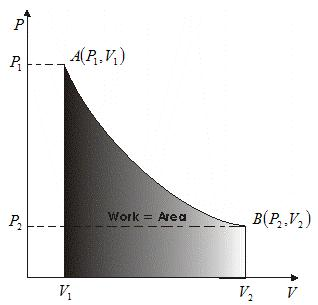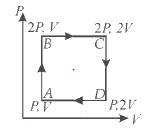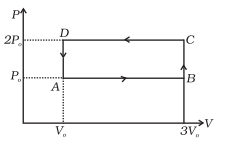# Work done by gas

## Work done by gas for Volume changes

• Consider a cylinder filled with gas and equipped with a movable piston as shown in fig belowfig - Force exerted by a system during small expansion.
Suppose,
A - Cross Sectional area of cylinder
P - Pressure exerted by piston at the piston face.
PA - Force exerted by the system.
• If piston moves out by a distance dx then Work done by this force is dW given by
$dW = PAdx$
$= PdV$ ---(1)
since $V = Adx$ and dV is change in volume of the system.
• In a finite volume change from V1 to V2
$W=\init PdV$ -----(2)
where limits of integration goes from V1 to V2
Graphically this relationship is shown below• Thus eqn (2) can be interpreted graphically as area under the curve between limits V1and V2.
• If pressure remains constant while the volume changes, then work is
W = P(V2-V1)(3)
• Work done not only depends on initial and final states but also on the intermediate states i.e., on the path.
Learning:Work done in a process is given by area under the process on the PV diagram

## Solved Example

Question 1
A ideal monatomic gas is taken through the process ABCDA as shown in below P-V diagram. Calculate the Work done by gasSolution
Work done by the gas from A to B = 0 ( as volume constant and area enclosed by AB is zero)
Work done by the gas from B to C = 2PV ( Area enclosed by line BC)
Work done by the gas from C to D = 0 ( as volume constant and area enclosed by AB is zero)
Work done by the gas from D to A = -PV ( Area enclosed by line BC and negative as volume is decreased)
Net Work Done = 2PV - PV = PV

Question 2
An ideal gas undergoes cyclic process ABCDA as shown in given P-V diagram .The amount of work done by the gas isSolution
Work done by the gas from A to B = 2PV ( Area enclosed by line AB)
Work done by the gas from B to C = 0 ( as volume constant and area enclosed by BC is zero)
Work done by the gas from C to D = -4PV ( Area enclosed by line CD and negative as volume is decreased)
Work done by the gas from D to A = 0 ( as volume constant and area enclosed by DA is zero)
Net Work Done = 2PV - 4PV = -2PV